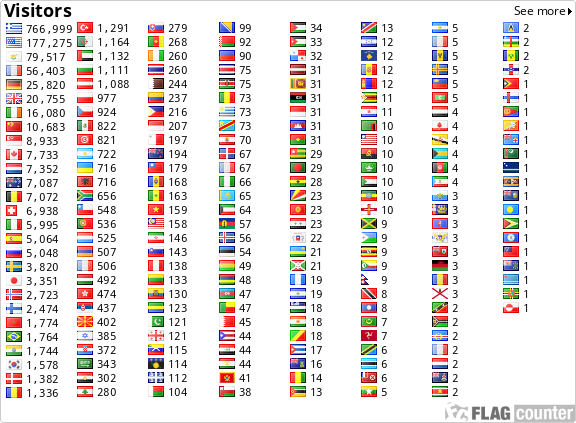﻿Réflexions méthodologiques en Calcul Formel

Réflexions méthodologiques en Calcul Formel

N. Lygeros

 Abstract: Methodological Reflections in Computer Algebra Abstract: We show, starting from some examples in Maple drawn from our teaching these last two years in first and second year DEUG in Lyon-I University and in Preparatory Classes (Math. Sup. and Math. Spé.) in the Lycee du Parc and Lycee of la-Martinière-Montplaisir, how computer algebra can be integrated in, and how it can change our relation to, the teaching of mathematics. We start by examining some common prejudices on the use of Maple. Then we approach various applications, elementary but rich in teaching (nature of the layout of a curve and study of the Fibonacci serie) while insisting on the necessity of an understanding of the working procedure of computer algebra ("black box" factorization). Then we analyze some fundamental differences which exist in the field of the lexicon between a low-level language and computer algebra. Finally we highlight, thanks to magic matrices exeample, the heuristic interest of formal approach. In appendix, we develop two more complex examples from the technical point of view, such as a problem of factorization and the fractal superinterpretation of Conway's commentaries. ArticleOpus